Murnaghan equation of state

The Murnaghan equation of state was developed by Francis D. Murnaghan of John Hopkins University. He presented an equation of state suitable for representing solids . Having high energy dependence on volume, the equation of state has found considerable use in condensed phase media.

Three derivative relations are utilised to lead to the formulation, namely: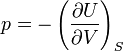$p=-\left(\frac{\partial U}{\partial V}\right)_S$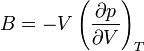$B=-V\left(\frac{\partial p}{\partial V}\right)_T$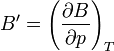$B'=\left(\frac{\partial B}{\partial p}\right)_T$

Where$p$ is the pressure,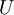$U$ is the internal energy,$V$ is the volume,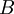$B$ is the bulk modulus and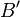$B'$ is the pressure derivative of the bulk modulus at constant temperature.

Making the assumption that$B'$ is constant allows one to assign a linear dependence of the bulk modulus on the pressure, which allows specifying one bulk modulus and derivative represented as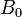$B_0$ and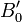$B_0'$ as the material constants. This leads to a relationship for pressure: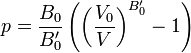$p=\frac{B_0}{B_0'}\left(\left(\frac{V_0}{V}\right)^{B_0'}-1\right)$

That can be integrated to represent the energy of the solid: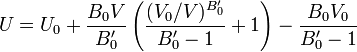$U=U_0+\frac{B_0V}{B_0'}\left(\frac{(V_0/V)^{B_0'}}{B_0'-1}+1\right)-\frac{B_0V_0}{B_0'-1}$

Regions of Applicability

Many papers have since been published on the applicability of the Murnaghan equation of state, many of which have presented alternate equation of state forms for solids. In general, it has been shown that the Murnaghan equation of state breaks down for compression ratios greater than ~0.7-0.8 times the original volume, which occurs as a consequence of the linear dependence of the bulk modulus on pressure and constant bulk modulus pressure derivative. Several popular forms presented to address this issue are the Birch-Murnaghan equation of state, the Vinet (Universal) equation of state, the Holzapfel equation of state, the Kumari-Dass equation of state, and the Baonza equation of state.